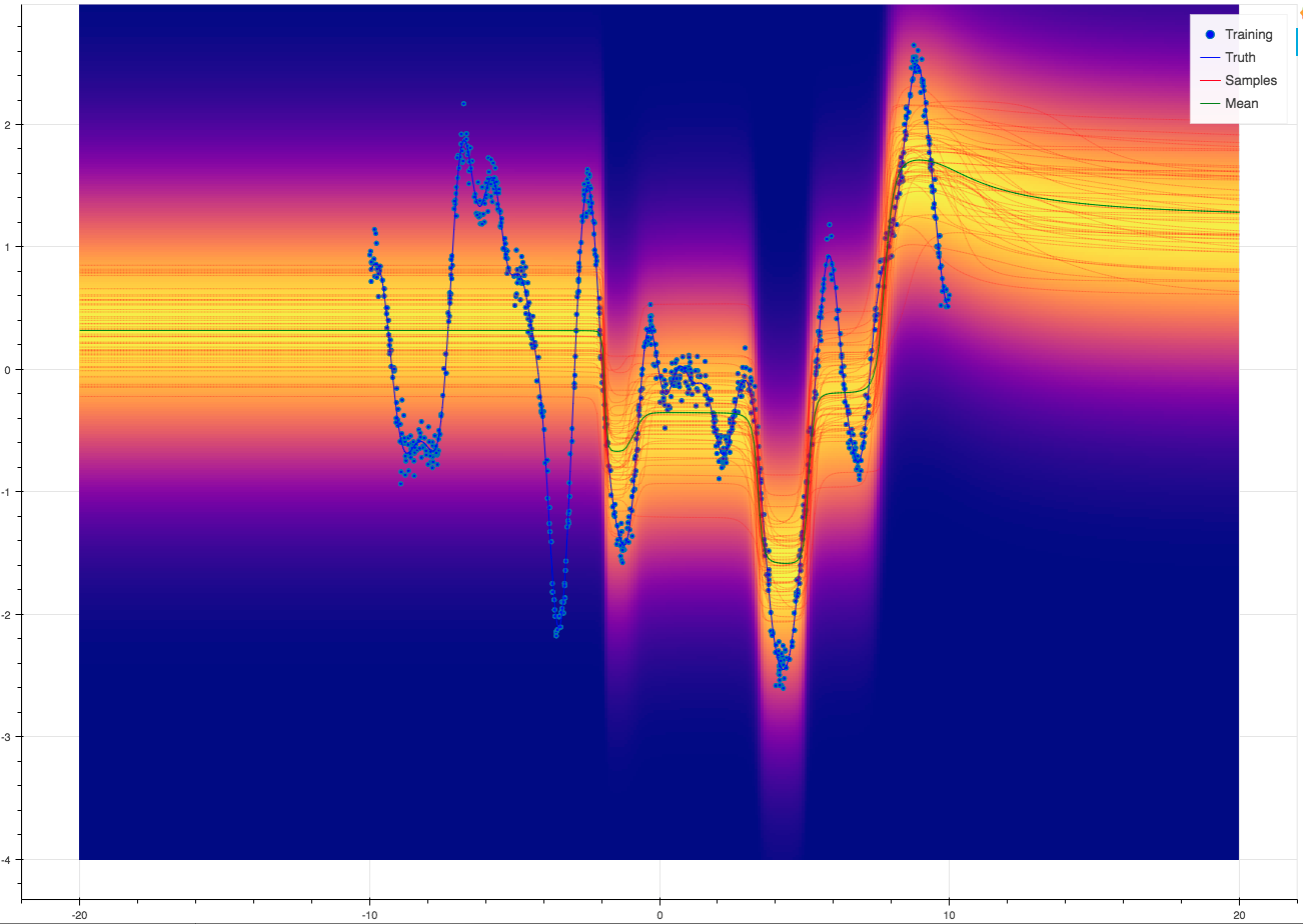# Integrating Aboleth with Keras¶

In most circumstances, Aboleth’s layer composition framework is interoperable with TensorFlow and Keras layers. This gives us access to a vast range of layers not directly implemented in Aboleth which are suitable for various problems, such as LSTMs, GRUs and other variants of recurrent layers for sequence prediction, to name just one example. Furthermore, this also allows us to readily take advantage of the various sophisticated normalization and activation layers proposed in the current research, such as Batch Normalization , Leaky ReLU, ELU , et cetera.

Here we define a simple wrapper layer that allows one to plug in Keras / TensorFlow layers.

class WrapperLayer(SampleLayer):

def __init__(self, layer, *args, **kwargs):

self.layer = layer(*args, **kwargs)

def _build(self, X):
"""Build the graph of this layer."""

# keras first flattens the n - len(input_shape) dimensions
# of the array and operates on last len(input_shape) dimensions
# which has shape input_shape
Net = self.layer(X)
# aggregate layer regularization terms
KL = tf.reduce_sum(self.layer.losses)

return Net, KL


Now we can use the wrapped layers and Aboleth’s native layers interchangeably. For example, consider tackling a toy regression problem using a deep neural net with dropout layers, where we perform maximum a posteriori (MAP) estimation of the layer weights / biases. The following are effectively equivalent:

net = (
ab.InputLayer(name="X", n_samples=n_samples_) >>
ab.DenseMAP(output_dim=64, l2_reg=0.01, l1_reg=0.) >>
ab.Activation(tf.tanh) >>
ab.DropOut(keep_prob=.5) >>
ab.DenseMAP(output_dim=64, l2_reg=0.01, l1_reg=0.) >>
ab.Activation(tf.tanh) >>
ab.DropOut(keep_prob=.5) >>
ab.DenseMAP(output_dim=1, l2_reg=0.01, l1_reg=0.)
)

l1_l2_reg = tf.keras.regularizers.l1_l2(l1=0., l2=0.01)

net = (
ab.InputLayer(name="X", n_samples=n_samples_) >>
WrapperLayer(tf.keras.layers.Dense, units=64, activation='tanh',
kernel_regularizer=l1_l2_reg, bias_regularizer=l1_l2_reg) >>
ab.DropOut(keep_prob=.5) >>
WrapperLayer(tf.keras.layers.Dense, units=64, activation='tanh',
kernel_regularizer=l1_l2_reg, bias_regularizer=l1_l2_reg) >>
ab.DropOut(keep_prob=.5) >>
WrapperLayer(tf.keras.layers.Dense, units=1, kernel_regularizer=l1_l2_reg,
bias_regularizer=l1_l2_reg)
)Now it’s easy to augment this model by incorporating other building blocks from Keras, e.g.

net = (
ab.InputLayer(name="X", n_samples=n_samples_) >>
WrapperLayer(tf.keras.layers.Dense, units=64) >>
WrapperLayer(tf.keras.layers.BatchNormalization) >>
WrapperLayer(tf.keras.layers.LeakyReLU) >>
ab.DropOut(keep_prob=.5) >>

WrapperLayer(tf.keras.layers.Dense, units=64) >>
WrapperLayer(tf.keras.layers.BatchNormalization) >>
WrapperLayer(tf.keras.layers.LeakyReLU) >>
ab.DropOut(keep_prob=.5) >>

WrapperLayer(tf.keras.layers.Dense, units=1, kernel_regularizer=l1_l2_reg,
bias_regularizer=l1_l2_reg)
)


Or use it to perform classification on sequences:

net = (
ab.InputLayer(name="X", n_samples=n_samples_) >>
WrapperLayer(tf.keras.layers.LSTM, units=128) >>
ab.DropOut(keep_prob=.5) >>
WrapperLayer(tf.keras.layers.Dense, units=1)
)


You can find the script here: regression_keras.py

  S. Ioffe and C. Szegedy, “Batch Normalization: Accelerating Deep Network Training by Reducing Internal Covariate Shift,” in Proceedings of the 32nd International Conference on Machine Learning, 2015, vol. 37, pp. 448–456.
  D.-A. Clevert, T. Unterthiner, and S. Hochreiter, Fast and Accurate Deep Network Learning by Exponential Linear Units (ELUs),” Nov. 2015.